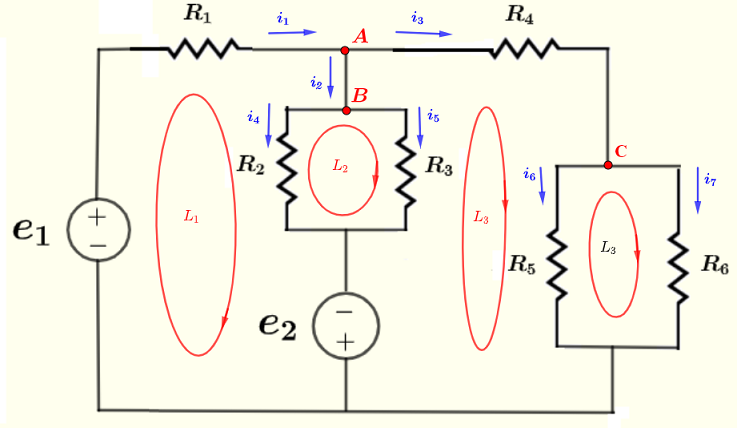# DC Kirchhoff's Equations Solver and Calculator

   
An online calculator to solve Kirchhoff's equations in a dc circuit is presented. The calculator accepts any number of equations. The outputs are the values of all currents in the given circuit.

## Write the voltages and Currents Equations Using Kirchhoff's

Example
Find all currents through the resistors in the circuit below, given the voltage sources $e_1 = 20$ V, $e_2 = 5$ V, and the resistances $R_1 = 100 \; \Omega$ , $R_2 = 120 \; \Omega$ and $R_3 = 60 \; \Omega$.step 1 - Use Kirchhoff's laws of voltages to write an equation for each cloed loop:
Loop L1: $e_1 - R_1 i_1 - R_2 i_2 + e_2 = 0$
Loop L2: $R_2 i_2 - R_3 i_3 = 0$
step 2: Use Kirchhoff's laws of currents to write an equation at each node:
Node A: $i_1 - i_2 - i_3 = 0$
step 2 - Rearrange the equations so that terms depending on the unknowns are on the left and all constants on the right, and order the unknowns $i_1, i_2$ and $i_3$ in each equation:
$\begin{array}{lclcl} R_1 i_1 + R_2 i_2 & = & e_1 + e_2 \\ R_2 i_2 - R_3 i_3 & = & 0 \\ i_1 - i_2 - i_3 & = & 0 \end{array}$
step 3 - Substitute all resistances of resistors and voltages of voltage sources by their numerical values and include all unknowns in the equations including the ones whose coefficents are zeros:
$\begin{array}{lclcl} 100 \; i_1 + 120 \; i_2 + 0 \; i_3& = & 25 \\ 0 \; i_1 + 120 i_2 - 60 i_3 & = & 0 \\ i_1 - i_2 - i_3 & = & 0 \end{array}$
step 4 - Enter the number of equations $m$ ( which is equal to the number of unknowns) and the coefficients of $i_1, i_2$ and $i_3$ into the calculator and find the currents:
Enter Number of Equations: $m =$

Change values of coefficients in above matrix (if needed) and click

## More Activities

Find all currents through the resistors in the circuit below, given the voltage sources $e_1 = 20$ V, $e_2 = 5$ V, and the resistances $R_1 = 100 \; \Omega$, $R_2 = 120 \; \Omega$, $R_3 = 60 \; \Omega$, $R_4 = 40 \; \Omega$, $R_5 = 240 \; \Omega$ and $R_6 = 80 \; \Omega$.Note: The circuit has 4 loops and 3 nodes which gives 7 equations with 7 unknowns $i_1, i_2 ,...., i_7$
The solution to this activity is in Solve DC Circuits Problems , problem 3.